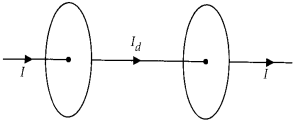Enlightened

# Question 1: NCERT Solutions for 12th Class Physics: Chapter 8-Electromagnetic Waves

• 0

Question 1: NCERT Solutions for 12th Class Physics: Chapter 8-Electromagnetic Waves

Figure shows a capacitor made of two circular plates each of radius 12 cm, and separated by 5.0 mm. The capacitor is being charged by an external source (not shown in the figure). The charging current is constant and equal to 0.15 A.(a) Calculate the capacitance and the rate of change of potential difference between the plates.
(b) Obtain the displacement current across the plates.
(c) Is Kirchhoff’s first rule (junction rule) valid at each plate of the capacitor? Explain.

Share

1. Solution:

(a) Capacitance of the parallel plate capacitor(b) The magnitude of displacement current is equal to conduction current. ∴ Id = I = 0.15 A
(c) Yes, Kirchhoff’s first law is very much applicable to each plate of capacitor as ld = I. So current is continuous and constant across each plate.Check the complete chapter with solutions.

NCERT Solutions for 12th Class Physics: Chapter 8-Electromagnetic Waves

• 0